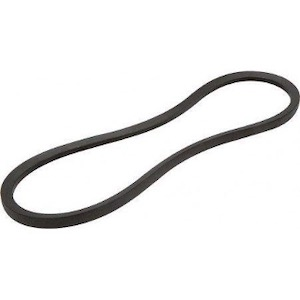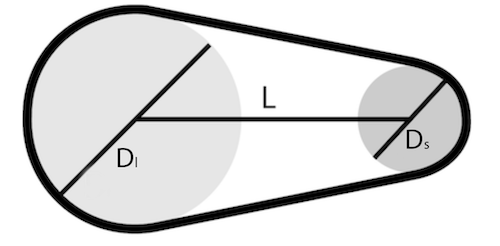# V-Belt Size CalculatorFigure 1: A v-belt

A v-belt is a transmission belt made of rubber polymer with a trapezoidal cross-section. These belts install around multiple groove pulleys for higher torque transmissions. Industrial motors, agricultural, textile machinery, and mining applications use v-belts. They are easy to select, simple to install, and offer years of reliable performance. This article discusses the basics of a v-belt and calculating the belt length of a two-pulley system. To learn more about v-belts, read our v-belt overview article.

## What is a v-belt pulley system?

A pulley is a wheel with one or more wedged grooves on its surface. It transfers motion and power using a cable or belt in its track. A belt drive is a popular power transmission method. It transmits power between multiple shafts using pulleys and elastic belts (e.g., v-belts).

A v-belt effectively links a power drive to a needed function. A v-belt drive’s typical application is for two pulleys near one another, which allows for compact construction. The wedge-shaped belt fits perfectly into the pulley’s wedged groove to create a matched fit. Due to this match, v-belts have tremendous gripping power, and they can transmit more force than flat belts.

The disadvantage of v-belts is efficiency. V-belts are about 2.5-3.0% less efficient than flat belts. Flat belts have this extra efficiency because they have a thin cross-section and, therefore, lower losses from bending.

## How to measure a v-belt length of a two-pulley system

Measuring v-belts is a common task to replace v-belts that show signs of wear and tear. Use the following formula as a v-belt length calculator for a v-belt in a two-pulley system with different pulley diameters:• Dl: Diameter of the larger pulley (mm, in)
• Ds: Diameter of the smaller pulley (mm, in)
• L: Distance between the pulleys’ axles (mm, in)

The above formula can also calculate the distance between the pulley axles if the v-belt length and pulley diameters are known.Figure 2: Diameters of a two-pulley system (Dl and Ds) and distance between the pulleys’ axles (L).

For example, consider calculating v-belt sizes in a factory. In one instance, the diameters of the pulleys are 200 mm and 120 mm, respectively, and the distance between the pulley axles is 1300 mm.

• Dl = 200 mm
• Ds = 120 mm
• L = 1300 mm

Substituting these values in the formula for belt length:=3.1m / 3100 mm

Sizing v-belts correctly for a pulley system is critical. If the chosen v-belt size is larger than the necessary value, the belt tends to be loose. A loose belt causes slippage along with wearing out of the pulleys. If the chosen length is less than the necessary value, excess tension and strain on the belt, shafts, and bearings cause premature wear of these components. Learn more by reading our v-belt tensioning article.

## Alternate formula to calculate belt length

Calculating v-belt length can be simpler. An approximation of the above formula is given below, which can also calculate the belt length in a two-pulley system:Where:

• Dl: Diameter of the larger pulley (mm, in)
• Ds: Diameter of the smaller pulley (mm, in)
• L: Distance between the axles of the pulleys (mm, in)

This equation for belt length approximates the original equation. It is convenient because it doesn’t hold complex computations like square roots or inverse trigonometric functions.

## V-belt length chart

When determining the size of a v-belt is a common task, it is useful to have a v-belt length chart on hand. The chart helps users understand the labels on a v-belt and quickly determine its length. Such a chart is available in our v-belt size chart article.

## FAQs

### How are v-belts measured?

If a v-belt is connected to a belt drive and the following is known: the diameter of each pulley and distance between pulleys, a v-belt is measured using an equation. If a v-belt is not on a pulley system, it can be measured using a measuring tape.

### How is the distance between two pulleys measured?

On a v-belt drive, if the diameter of each pulley is known, as well as the length of the v-belt, the distance between the pulley axles is calculated using a formula.